# Using ANOVA to Find Differences in Population Means

By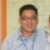Chew Jian Chieh

Three methods used to dissolve a powder in water are compared by the time (in minutes) it takes until the powder is fully dissolved. The results are summarized in the following table: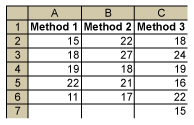It is thought that the population means of the three methods m1, m2 and m3 are not all equal (i.e., at least one m is different from the others). How can this be tested?

One way is to use multiple two-sample t-tests and compare Method 1 with Method 2, Method 1 with Method 3 and Method 2 with Method 3 (comparing all the pairs). But if each test is 0.05, the probability of making a Type 1 error when running three tests would increase.

A better method is ANOVA (analysis of variance), which is a statistical technique for determining the existence of differences among several population means. The technique requires the analysis of different forms of variances – hence the name. But note: ANOVA is not used to show that variances are different (that is a different test); it is used to show that means are different.

### How ANOVA Works

Basically, ANOVA compares two types of variances: the variance within each sample and the variance between different samples. The following figure displays the data per method and helps to show how ANOVA works. The black dotted arrows show the per-sample variation of the individual data points around the sample mean (the variance within). The red arrows show the variation of the sample means around the grand mean (the variance between).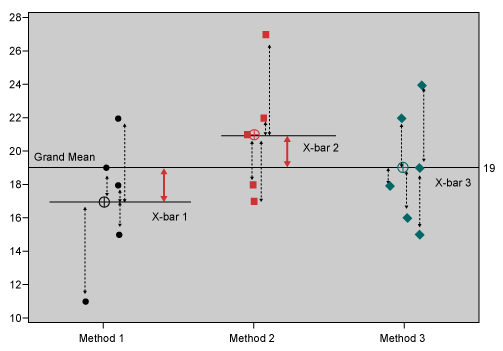Comparing Variances Using ANOVA

The assumption is: If the population means are different, then the variance within the samples must be small compared to the variance between the samples. Hence, if the variance between divided by the variance within is large, then the means are different.

Handpicked Content:   When Does a Difference Matter? Using ANOVA to Tell

### Steps for Using ANOVA

Step 1: Compute the Variance Between

First, the sum of squares (SS) between is computed: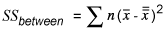Where x-bar is the sample mean and x-double-bar is the overall mean or grand mean. This can be easily found using spreadsheet software: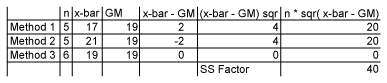Now, the variance between or mean square between (ANOVA terminology for variance) can be computed.

The formula for sample variance is: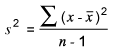Since there are three sample means and a grand mean, however, this is modified to: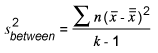Where k is the number of distinct samples. In other words, the variance between is the SS between divided by k – 1: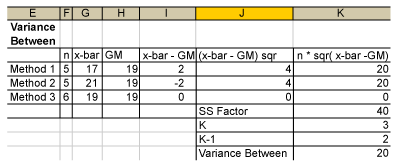(This example uses Microsoft Excel software. In Minitab software, SS between is called SS factor, variance between is called MS factor and K – 1 is called DF.)

Step 2: Compute the Variance Within

Again, first compute the sum of squares within. This is: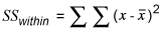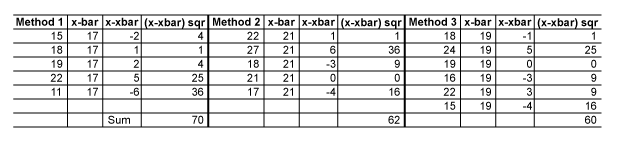SS within is 70 + 62 + 60 = 192.

To obtain the variance within, use this equation: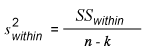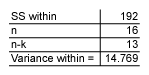Step 3: Compute the Ratio of Variance Between and Variance Within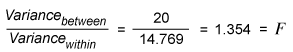This is called the F-ratio. How can this be interpreted? If the null hypothesis is true, meaning m1, m2 and m3 are all equal, then the variance between the samples is 0 (zero) (i.e., the F-ratio is also zero).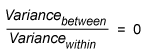If the null hypothesis is not true, then this F-ratio will become larger, and the larger it gets, the more likely it is that the null hypothesis will be rejected.

Handpicked Content:   How To Compare Data Sets – ANOVA

Using the F-tables for k = 3 and n = 16, one gets a p-value of 0.292 (use the FDIST function in Excel). This means that the probability that the observed F-ratio of 1.354 is random is 29.2 percent: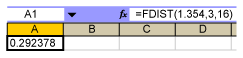Hence, if one sets a = 0.05, one must accept the null hypothesis that there is no difference in the population means.

In Minitab, the results for the same data are displayed in the session window like this: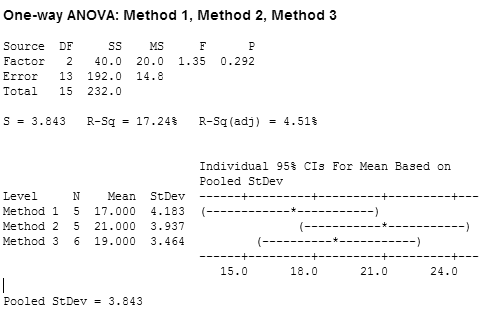If there had been a significant difference between the samples, this would have been seen with the p-value and also there would have been at least one confidence interval for one mean that had no, or very little, overlap with the other confidence intervals. This would have indicated a significant difference between its population mean and the other population means.

Minitab also computes an R-squared value (R-Sq) by taking the SS factor/SS total = 40/232 *100 = 17.24. This shows the percent of explained variation by the factor. Here, the factor only explains 17.24 percent of the total variation; hence, it is not a very good explanation.

##### Chew Jian Chieh

1.Irfan

Its a good and simple way of providing the basic concepts of ANOVA. Please do keep on providing more info using real life examples and use of ANOVA and explain how the problem was solved.

2.Sara

This article was very helpful! Coming from someone who has never used ANOVA before or know what it’s for, I feel like I can teach someone else now. Thanks!

3.saba bashir

My experiance with this article is very fruitfull. This is an easy to understand the whole concept of ANOVA.After reading this my concepts about ANOVA has become more clear.Really its a good job.Thanks

4.Erik Laufer

I posted these comments against a link posted in LinkedIn. I’m copying them over here to see if there’s more context behind the case study that was shared and how to more effectively capitalize on comparative experiments that could lead to more formal active experimentation….

The mechanics of the ANOVA example linked above does a pretty decent job of running one through the mechanics of the ANOVA platform itself. However, we must place ANOVA in the greater context of Hypothesis Testing. The miss that I see in the example is that the 4 steps of any hypothesis test were not rigorously followed and the important caveat that is written in invisible ink above the gates of any Six Sigma class. To do a hypothesis test requires that the data being assessed is stable/predictable and free of OOC/OOT signals…. 1. Practically State the Problem…this was done effectively by stating that we wanted to test for the difference in the population mu in one of the formulations. However, to make it more real world, and business impacting, there should have been a check in with SMEs/Process Owner/Customer to see what is the ‘practical difference in the mu’ that would constitute a potential signal of change that we actually care about and impacts the patient/customer. From here, given initial information from data collected/SPC we could proceed forward into a Power Study to generate minimum sample sizes required to fuel the study of interest… 2. Statistically State the Problem. This would be to transform our practical statement into its constituent Ho and Ha statements. Practically there should have been a check in to determine if any change is specifically of business interest…do we want to push the mu higher? Lower? etc… 3. Statistically Derive a Conclusion. There are two hurdles to pass here…1st, did we see a Statistical Signal of change? That is traditionally taken as an ANOVA result that generates a p value below the initial level of statistical significance selected. Typically that value is 0.05. But, it does not need to always be that….again that is a discussion between the experimenter and the SMEs. What’s the risk of being wrong? Are we dabbling our toes into something new? Could our estimates of expected delta and/or variation within the design space be too low/high? All of these would go to making a much more informed determination of the level that flags the level of statistical significance to reject the null. 4. Practically State a Conclusion. The 2nd hurdle is to demonstrate that we have met the practical delta of interest in the mu. Tukey’s HSD should have been consulted here to specifically address the issue of the statistical and practical significance attained. At this point, since a power study was not conducted, we should have conducted a post-power study given a delta observed of 2 units against an estimate of variability/noise of 3.843 units. Given that what was the power? Did we see any practical signals of interest that we could carry forward into a follow on DOE/comparative analysis? Kind regards, Erik

5.Miss I

Thank you so much for this very useful article. Was looking everywhere for a very simple and easy to understand explanation of ANOVA. And the use of terms appearing in MINITAB were also extremely useful. Wis the article also included how to get all these results using MINITAB. Thanks a lot :)

6.Can

How will we find which sample group is significantly different from which other sample group?

7.camila

This article was very awesome! I got much information about anova, by this article i cleared my concept.

8.Vythi

Found the ANOVA article very useful to understand concepts and mechanics. Tks!